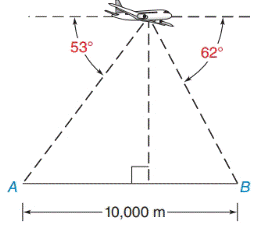Chapter 11.4, Problem 31E### Elementary Geometry for College St...

6th Edition
Daniel C. Alexander + 1 other
ISBN: 9781285195698

#### Solutions

Chapter
Section### Elementary Geometry for College St...

6th Edition
Daniel C. Alexander + 1 other
ISBN: 9781285195698
Textbook Problem
1 views

# In Exercises 29 to 34, use the Law of Sines or the Law of Cosines to solve each problem. Angle measures should be found to the nearest degree and areas and distances to the nearest tenth of a unit.A surveillance aircraft at point C sights an ammunition warehouse at A and enemy head-quarters at B through the angles indicated. If points A and B are 10, 000 m apart, what is the distance from the aircraft to enemy headquarters?To determine

To find:

The distance from the aircraft to enemy headquarters.

Explanation

Formula:

The Law of sines,

sinαa=sinβb=sinγc

Where α, β, and γ is the angle of the triangle, and a, b, and c is the sides of the triangle.

Calculation:

Given,

A surveillance aircraft sights an ammunition warehouse at A and enemy headquarters at B

They are 10, 000m apart, and their angles of observation are 53 and 62 respectively..

Let A and B were warehouse and enemy headquarters respectively and C is the surveillance aircraft with angle α.

Then, AB¯=10,000m, BC¯=x , B=53 , and A=62

a=10,000 m , b=x , β=53 , and γ=62

Find x:

For any triangle,

α+β+γ=180

Here,  β=53  and  γ=62

α+53+62=180

α+115=180

### Still sussing out bartleby?

Check out a sample textbook solution.

See a sample solution

#### The Solution to Your Study Problems

Bartleby provides explanations to thousands of textbook problems written by our experts, many with advanced degrees!

Get Started